##### Question

In: Statistics and Probability

# ACT and SAT scores are both known to be normally distributed. In 2017, the mean and...

ACT and SAT scores are both known to be normally distributed. In 2017, the mean and standard deviation for the ACT were μ=20 and σ=6, respectively. The mean and standard deviation for the SAT were μ=1060 and σ=200, respectively. a. What ACT score would place a student in the same percentile as a student who scored 1310 on the SAT in 2010? (In other words, what ACT score is "equivalent" to an SAT score of 1310?) Round your answer to the nearest integer. b. What SAT score would place a student in the same percentile as a student who scored 14.6 on the ACT in 2010? (In other words, what SAT score is "equivalent" to an ACT score of 14.6?) Round your answer to the nearest integer.

## Solutions

##### Expert Solution

Solution:

Given: ACT and SAT scores are both known to be normally distributed.

For the ACT :

μ=20 and σ=6.

For the SAT:

μ=1060 and σ=200.

Part a. What ACT score would place a student in the same percentile as a student who scored 1310 on the SAT in 2010?

x = 1310 for SAT

Find  z score for x = 1310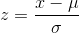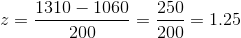Now use this z score value to find corresponding ACT score:

Use following formula to find x value ( ACT score):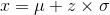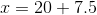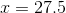ACT score would place a student in the same percentile as a student who scored 1310 on the SAT in 2010 is 28

Part b) What SAT score would place a student in the same percentile as a student who scored 14.6 on the ACT in 2010?

x = 14.6 for ACT.

Find  z score for x = 14.6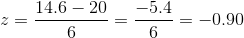Now use this z score value to find corresponding SAT score:

Use following formula to find x value ( SAT score):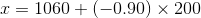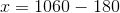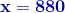Thus SAT score would place a student in the same percentile as a student who scored 14.6 on the ACT in 2010 is 880.

## Related Solutions

##### 33. 2017 SAT scores All sections of the test are known to be normally distributed with...
33. 2017 SAT scores All sections of the test are known to be normally distributed with mean of approximately mean of 1060 and standard deviation of 195 (a) Draw a normal curve with the parameters labeled. (b) Shade the region that represents the proportion of test takers who scored less than 1200. (c) Suppose the area under the normal curve to the left of X = 1200 is 0.7636. Provide two interpretations of this result.
##### 3)   Scores on the SAT are normally distributed with a mean of 500 and a standard...
3)   Scores on the SAT are normally distributed with a mean of 500 and a standard deviation of 100 What is the probability of obtaining a score greater than 640? What is the probability of obtaining a score less than 390? What is the probability of obtaining a score between 725 and 800? What is the probability of obtaining a score either less than 375 or greater than 650? 4) If you obtained a score of 75 on an test,...
##### The SAT scores for students are normally distributed with a mean of 1000 and a standard...
The SAT scores for students are normally distributed with a mean of 1000 and a standard deviation of 200. What is the probability that a sample of 45 students will have an average score between 970 and 1010? Round your answer to 3 decimal places.
##### SAT verbal scores are normally distributed with a mean of 430 and a standard deviation of...
SAT verbal scores are normally distributed with a mean of 430 and a standard deviation of 120 (based on data from the College Board ATP). If a sample of 35 students are selected randomly, find the probability that the sample mean is above 470. A.) 0.244 B.) 0.9756 C.) 0.0445 D.) 0.0244
##### Suppose that SAT math scores are normally distributed with a mean of 516 and a standard...
Suppose that SAT math scores are normally distributed with a mean of 516 and a standard deviation of 115, while ACT math scores have a normal distribution with a mean of 22 and a standard deviation of 5. James scored 650 on the SAT math and Jacob scored 29 on the ACT math. Who did better in terms of the standardized z-score? Group of answer choices James Jacob They did relatively the same. Impossible to tell because of the scaling
##### 31. The 2018 Math Portion of the SAT scores are normally distributed with a mean of...
31. The 2018 Math Portion of the SAT scores are normally distributed with a mean of 527 and a standard deviation of 117. Give the range of scores that will satisfy the 68%, 95% and 99.7% of the Empirical Rule. 32. Use the mean and standard deviation from #31 for the Math Portion of the SAT. Suzette took the SAT in 2018 and scored higher, on the Math Portion, than the bottom 30% but lower than the top 12% of...
##### The SAT scores for US high school students are normally distributed with a mean of 1500...
The SAT scores for US high school students are normally distributed with a mean of 1500 and a standard deviation of 100. 1. Calculate the probability that a randomly selected student has a SAT score greater than 1650. 2. Calculate the probability that a randomly selected student has a SAT score between 1400 and 1650, inclusive. 3. If we have random sample of 100 students, ﬁnd the probability that the mean scores between 1485 and 1510, inclusive.
##### The SAT scores earned on the math portion are approximately normally distributed with mean 516 and...
The SAT scores earned on the math portion are approximately normally distributed with mean 516 and standard deviation 116. Answer the following questions based on this information. Make sure you show your work. 1. Using a printout of the graph above or making your own copy, mark the mean SAT score on the math proportion at the appropriate place. Then mark values of score that are 1, 2, and 3 standard deviations away from the mean value. Labeling your marks...
##### Scores on the SAT Mathematics test are believed to be normally distributed. The scores of a...
Scores on the SAT Mathematics test are believed to be normally distributed. The scores of a simple random sample of five students who recently took the exam are 570, 620, 710, 540 and 480. We want to find a 95% confidence interval of the population mean of SAT math scores. A) Calculate the point estimate. (Round to four decimal places as​ needed.) B) Calculate the sample standard deviation. (Round to four decimal places as​ needed.) C) Calculate the standard error...
##### IQ scores are known to be normally distributed. The mean IQ score is 100 and the...
IQ scores are known to be normally distributed. The mean IQ score is 100 and the standard deviation is 15. What percent of the population has an IQ between 85 and 105. Need to solve it through Excel